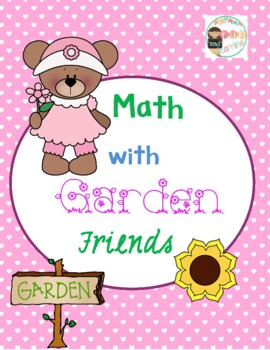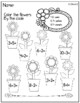# Garden Bear MathSubject
Resource Type
File Type
PDF (1 MB|7 pages)
Standards
FREE
• Product Description
• Standards
With these spring activities your children will enjoy practicing addition and subtraction facts. An activity for decomposing numbers to 19 is also included in this free packet.
Fluently add and subtract within 5.
Decompose numbers less than or equal to 10 into pairs in more than one way, e.g., by using objects or drawings, and record each decomposition by a drawing or equation (e.g., 5 = 2 + 3 and 5 = 4 + 1).
Compose and decompose numbers from 11 to 19 into ten ones and some further ones, e.g., by using objects or drawings, and record each composition or decomposition by a drawing or equation (e.g., 18 = 10 + 8); understand that these numbers are composed of ten ones and one, two, three, four, five, six, seven, eight, or nine ones.
Total Pages
7 pages